March 18, 2021

## Testing Causal Claims

• definition
• attributes
• problems

### 2. Problems with Correlation

• Random correlation
• Bias in correlation (confounding)

## Solving FPCI

We solve the fundamental problem of causal inference by:

• comparing the values of the outcome ($$Y$$) across cases we can observe with different values of the cause ($$X$$)
• assuming that cases with different values of $$X$$ can stand in for the same case in the counterfactual world where its value of $$X$$ is different.

## Correlation

Correlation is the degree of association/relationship between the observed values of $$X$$ (the independent variable) and $$Y$$ (the dependent variable)

• There are formal mathematical definitions.
• We use the term loosely to describe observed relationship between $$X$$ and $$Y$$

## Correlation

All empirical evidence for causal claims relies on correlation between the independent and dependent variables.

But, you’ve all heard this: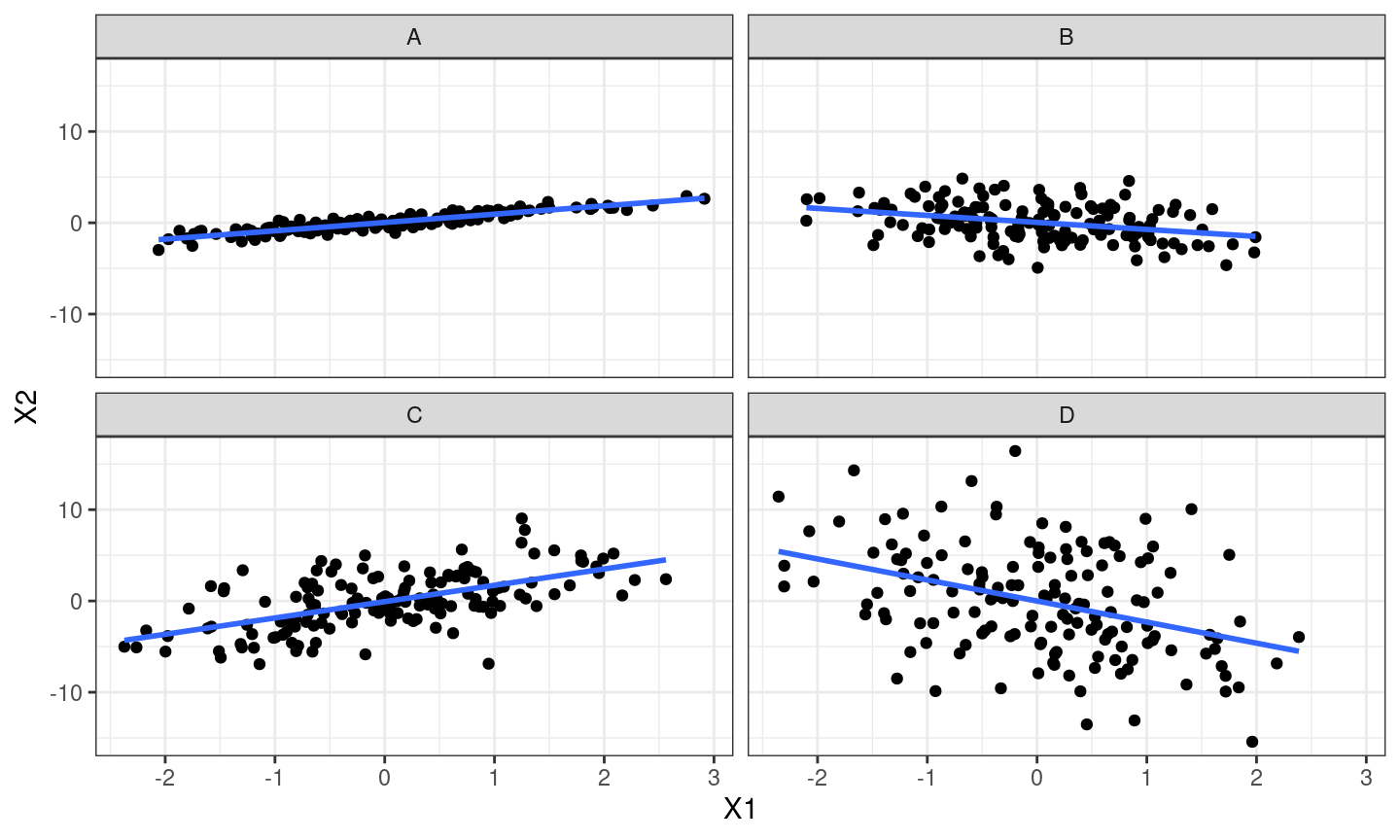POLL

## Correlation

Correlations have

• direction:
• positive: implies that as $$X$$ increases, $$Y$$ increases
• negative: $$X$$ increases, $$Y$$ decreases
• strength (has nothing to do size of effect):
• strong: $$X$$ and $$Y$$ almost always move together (near $$1,-1$$)
• weak: $$X$$ and $$Y$$ do not move together very much (near $$0$$)
• slope/effect size:
• this is the how much $$Y$$ changes with $$X$$.
• The larger the effect of $$X$$ on $$Y$$, the steeper the slope

## Correlation

What do we need to assume to use correlation as evidence of causation?

### Two types of problems

• random association: correlations between $$X$$ and $$Y$$ occur by chance and do not reflect causal relationship.

• bias (spurious correlation, confounding): $$X$$ and $$Y$$ are correlated but the correlation does not result from a causal relationship between those variables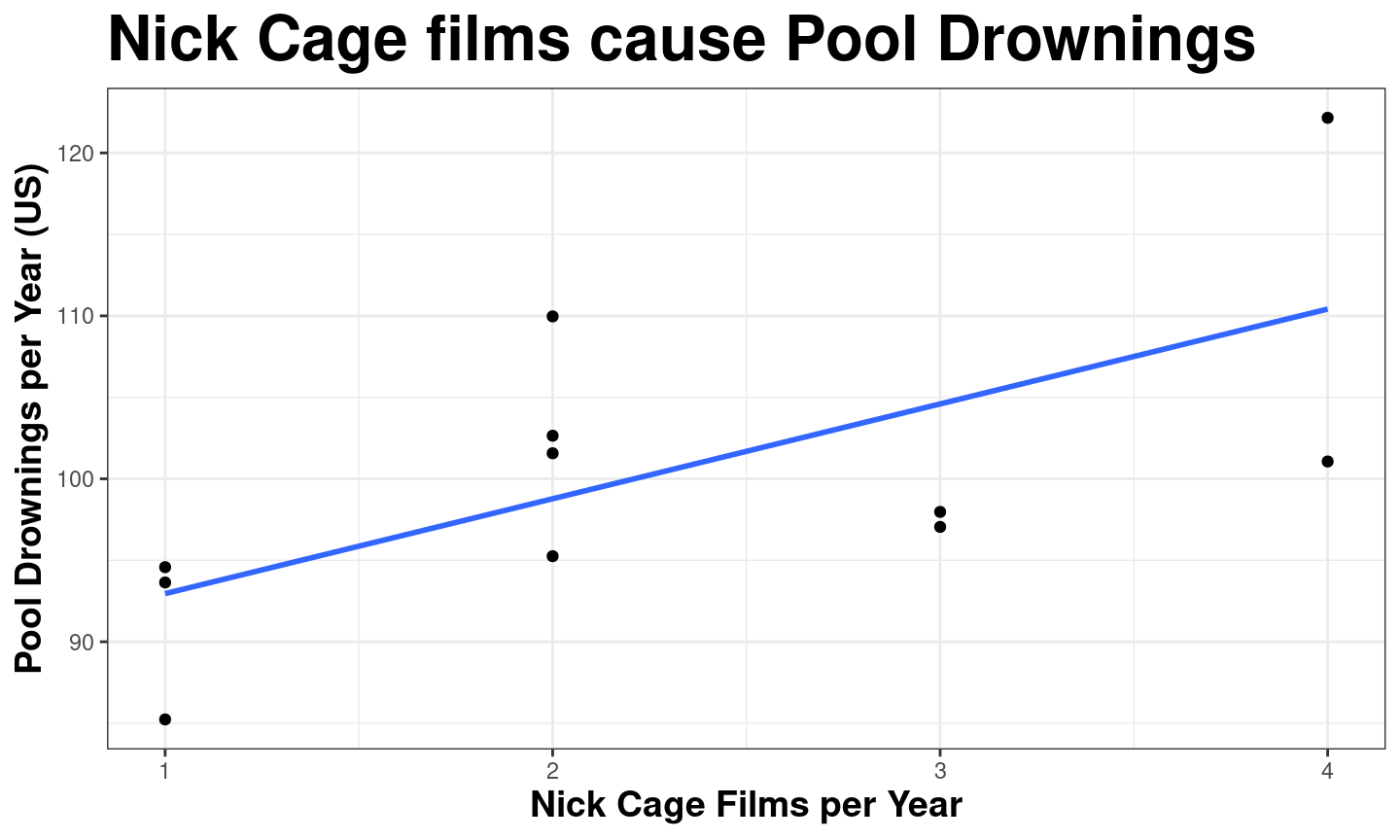## Correlation: Random association

How do we know a correlation is systematic?

• How do we know that it is not simply a pattern by random chance?
• Apparent patterns can be produced by pure randomness

## Correlation: Random association

If you look at enough possible sets of variables, you might find a strong correlation

• But it could have happened by chance!
• So a correlation might not be meaningful (e.g. Nick Cage)

(Arbitary Correlations)[http://www.tylervigen.com/spurious-correlations]

## Random association: Statistics

To see that random patterns can emerge, I use random number generators to

• randomly pick $$5$$ values of $$X$$
• randomly pick $$5$$ values of $$Y$$

We can imagine these are the observed $$X$$ and $$Y$$ for $$5$$ cases.

How easy is it to find a strong correlation?

## Random association: Statistics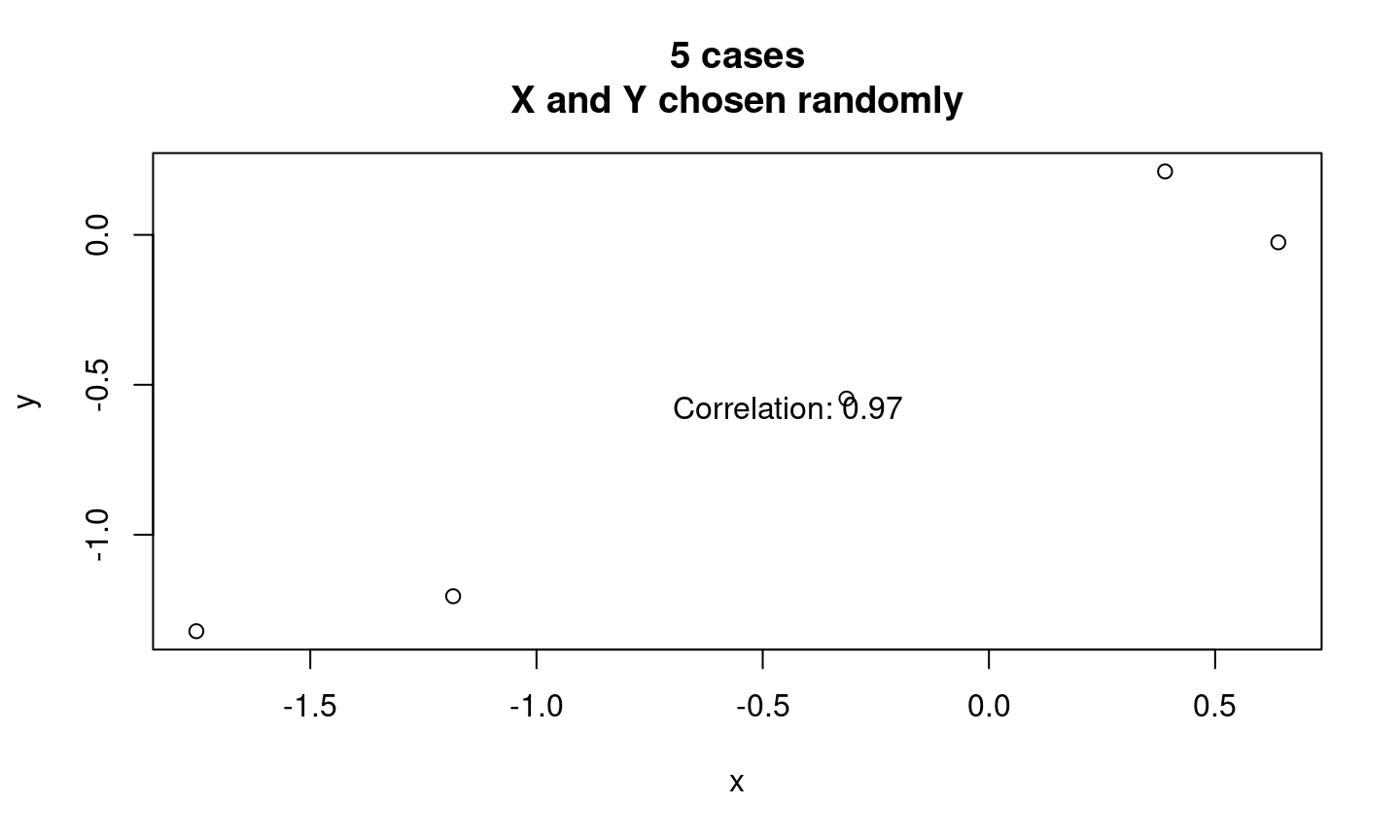## Random association: StatisticsTries to get correlation $$> 0.9$$: 1

## Random association: Statistics

Field of statistics investigates properties of chance events (stochastic processes):

• Probability theory tells us how likely events are to happen, given chance
• Can tell us how likely correlation of some value is to happen by chance

## Random association: Statistics

### How?

1. Compute correlation of $$X$$ and $$Y$$
2. How strong is the correlation>
• Patterns that are stronger are less likely to happen by chance
3. How many cases do we have?
• Patterns with many cases are less likely to happen by chance
4. Assign a probability that the correlation we see would have happened by chance

## Random association: Statistics

This procedure works…

### Assuming…

we know the chance processes that might affect this correlation

## Random association: Statistics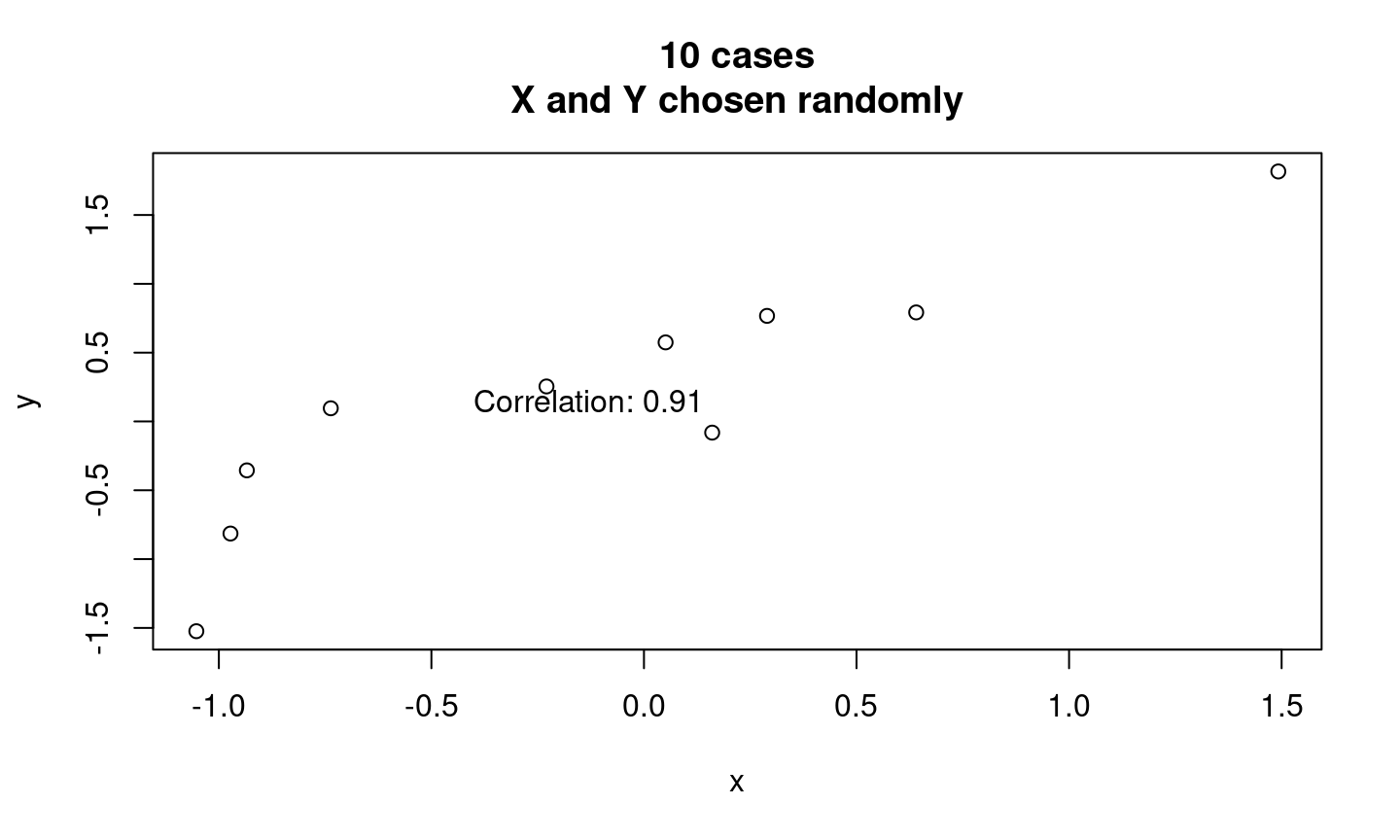Tries to get correlation $$> 0.9$$: 397

## Random association: Statistics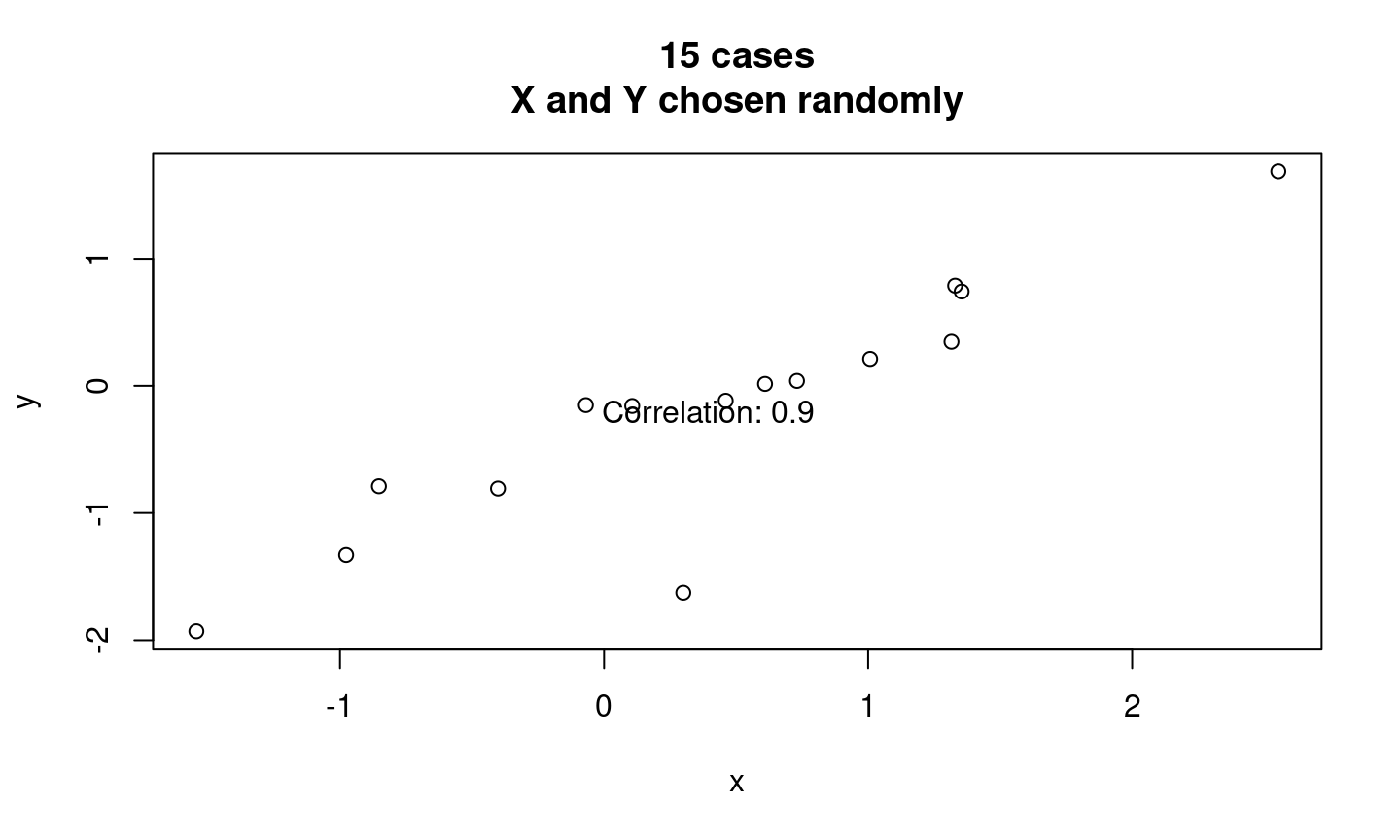Tries to get correlation $$> 0.9$$: 63963

## Random association: Statistics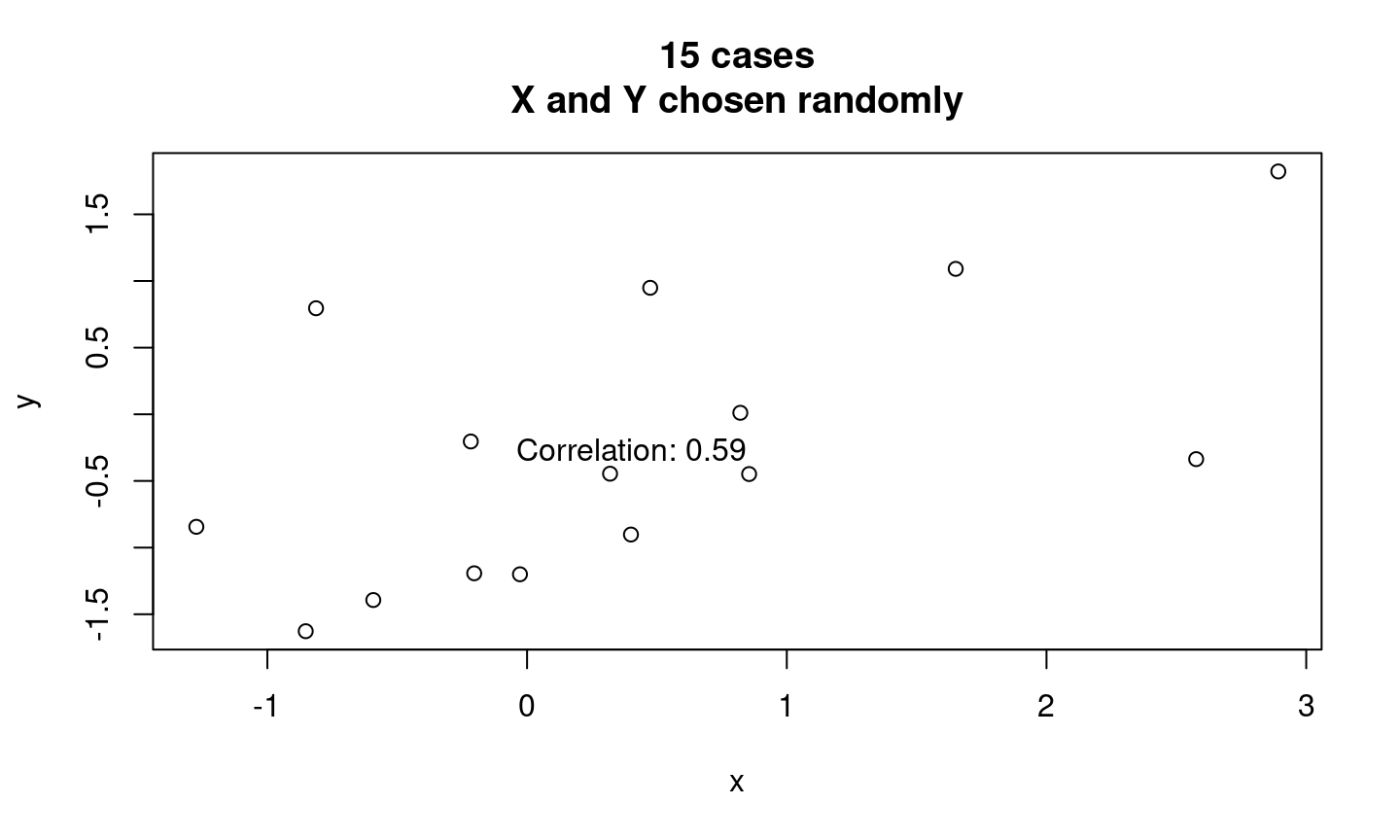Tries to get correlation $$> 0.45$$: 76

## Random association: Statistics

statistical significance:

An indication of how likely correlation we observe could have happened purely by chance.

higher degree of statistical significance indicates correlation is unlikely to have happened by chance

## Random association: Statistics

$$p$$ value:

• A numerical measure of statistical significance. Puts a number on how likely observed correlation would have occurred by chance, assuming a we know the chance procedure and the truth is a $$0$$ correlation.

• It is a probability, so is between $$0$$ and $$1$$.

• Lower $$p$$-values indicate greater statistical significance

$$p < 0.05$$ often used as threshold for “significant” result.

• but it is not a magic number
• Can observe $$p < 0.05$$ by chance ($$\frac{1}{20}$$)

## Random association: Statistics

$$p$$ value:

Be wary of “$$p$$-hacking”

• $$p$$ values become meaningless if we look at many associations, then only report the ones that are “significant”.

### Why?

• low $$p$$-values occur by chance when we look at lots of associations

## Significant?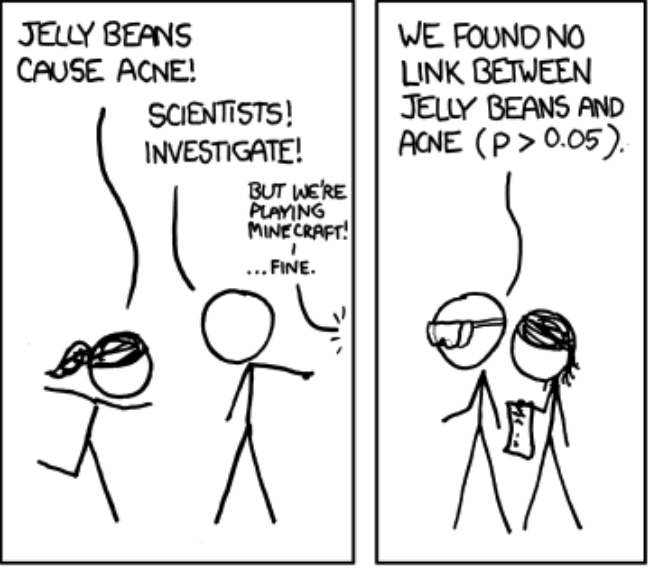## Significant?